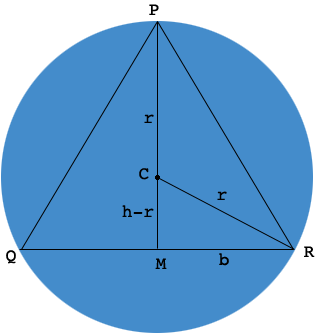SEARCH HOMEMath Central Quandaries & QueriesQuestion from Patrick: I'm trying to find a quick calculation to find the center of the circle that will touch the 3 points of an isosceles triangle.
Known dimensions are length of the triangle base and the height from the top point to the middle of the base.
Kind regards,
PatrickHi Patrick,

In my diagram the center $C$ of the circle is between the top point $P$ and the middle $M$ of the base.Let the radius of the circle be $r$ and then $|PC| = |CR| = r.$ If $h$ is the height of the triangle then $|CM| = h-r.$ Let the distance from $M$ to $R$ be $b,$ which is half the length of the base of the triangle. Triangle $PMR$ is a right triangle. Use Pythagoras theorem for this triangle and solve for $r.$

Apply a similar argument to find $r$ if $M$ is between $P$ and $C.$

PennyMath Central is supported by the University of Regina and The Pacific Institute for the Mathematical Sciences.